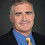## Thursday, September 02, 2021

### The hierarchy and GapP

There is a great but little-known theorem from the early 90's by Seinosuke Toda and Mitsunori Ogihara (buried as Lemma 2.3 in their paper) that shows the polynomial-time hierarchy is randomly low for Gap-P.

Let M be a non-deterministic polynomial-time machine. #M(x) is the number of accepting paths of M on x. #P is the set of functions f such that f(x) = #M(x) for an NP machine M.

Gap-M(x) is the difference between the number of accepting and rejecting paths. Unlike #M(x), Gap-M(x) could be negative. Gap-P is the set of functions such that f(x)=Gap-M(x) for an NP machine M. Equivalently Gap-P is the difference of two #P functions. Gap-P inherits all the nice closure properties of #P while being closed under negation and subtraction.

Theorem (Toda-Ogihara): Let q be a polynomial and f be a function in Gap-PPH. There is a function g in Gap-P and a polynomial p such that for all x, if you choose a binary string r randomly of length p(|x|), f(x) = g(x,r) with probability at least 1-2-q(|x|).

In other words, with randomness the PH as an oracle of Gap-P disappears.

Let me show you how the proof works for a specific f in GapPPH, namely the indicator function for SAT: f(φ) = 1 if φ is satisfiable and 0 otherwise.

Recall Valiant-Vazirani showed how to randomly reduce a formula φ to a set of formula ψ1,…,ψk such that

1. If φ is not satisfiable then for all i, ψi will not be satisfiable
2. If φ is satisfiable then with high probability for some i, ψi will have exactly one satisfying assignment.
Picking k > 4|x|q(|x|) will reduce the error in (2) to under 2-q(|x|). Let g(φ,r) use r to choose ψ1,…,ψk and output the Gap-P function 1-∏(1-#ψi) using the closure properties of Gap-P where #ψi is the number of satisfying assignments to ψi.
• If φ is not satisfiable then for all i, #ψi=0 and g(φ,r) = 0.
• If φ is satisfiable then with high probability for some i, #ψi=1 and thus g(φ,r) = 1.
Note that if r is a bad random sequence and φ is satisfiable, g(φ,r) might be an exponentially large integer, positive or negative. It's right most of the time but when it's wrong it's terribly wrong.

1.Does derandomization of Valiant Vazirani derandomize the full result or only this example?

1.Great question. The proof of the full Toda-Ogihara result requires a version of Valiant-Vazirani relative to the polynomial-time hierarchy and thus a stronger derandomization assumption. An assumption like DTIME(2^n) not in DSPACE(2^{o(n)}) will give you a PRG sufficient to fully derandomize Toda-Ogihara.

2.Can there be a converse such as deterministic Toda-Ogihara implies the time space separation? Or is it possible results such as EXP in PSPACE abd deterministic Toda-Ogihara are both simultaneously possible as far as we know?

1.Probably not a full converse but it would be interesting to see if there is some circuit lower bound one could obtain from a deterministic version of Toda-Ogihara. I'll leave that as an open question.

3.While on the question of derandomizing [VV85], here is a fine question: Is there a Pseudo-deterministic RP reduction from SAT to USAT, i.e., the reduction can use random bits but reruns of the reduction should produce the same instance of USAT with very high probability.